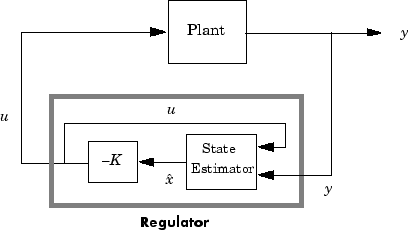Documentation

# reg

Form regulator given state-feedback and estimator gains

## Syntax

```rsys = reg(sys,K,L) rsys = reg(sys,K,L,sensors,known,controls) ```

## Description

`rsys = reg(sys,K,L) ` forms a dynamic regulator or compensator `rsys` given a state-space model `sys` of the plant, a state-feedback gain matrix `K`, and an estimator gain matrix `L`. The gains `K` and `L` are typically designed using pole placement or LQG techniques. The function `reg` handles both continuous- and discrete-time cases.

This syntax assumes that all inputs of `sys` are controls, and all outputs are measured. The regulator `rsys` is obtained by connecting the state-feedback law u = –Kx and the state estimator with gain matrix `L` (see `estim`). For a plant with equations

`$\begin{array}{l}\stackrel{˙}{x}=Ax+Bu\\ y=Cx+Du\end{array}$`

this yields the regulator

`$\begin{array}{l}\stackrel{˙}{\stackrel{^}{x}}=\left[A-LC-\left(B-LD\right)K\right]\stackrel{^}{x}+Ly\\ u=-K\stackrel{^}{x}\end{array}$`

This regulator should be connected to the plant using positive feedback.`rsys = reg(sys,K,L,sensors,known,controls) ` handles more general regulation problems where:

• The plant inputs consist of controls u, known inputs ud, and stochastic inputs w.

• Only a subset y of the plant outputs is measured.

The index vectors `sensors`, `known`, and `controls` specify y, ud, and u as subsets of the outputs and inputs of `sys`. The resulting regulator uses [ud ; y] as inputs to generate the commands u (see next figure).## Examples

Given a continuous-time state-space model

```sys = ss(A,B,C,D) ```

with seven outputs and four inputs, suppose you have designed:

• A state-feedback controller gain `K` using inputs 1, 2, and 4 of the plant as control inputs

• A state estimator with gain `L` using outputs 4, 7, and 1 of the plant as sensors, and input 3 of the plant as an additional known input

You can then connect the controller and estimator and form the complete regulation system by

```controls = [1,2,4]; sensors = [4,7,1]; known = ; regulator = reg(sys,K,L,sensors,known,controls) ```